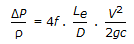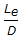# Chemical Engineering - Process Equipment and Plant Design

### Exercise :: Process Equipment and Plant Design - Section 4

1.

In common bubble cap distillation column design practice, riser area is approximately equal to (where, Aa = Annular passage area, and Sa = Slot area)

 A. Aa = Sa B. 1.2 Aa = 1.2 Sa C. Sa = 1.5 Aa D. Aa = 1.5 Sa

Answer: Option A

Explanation:

No answer description available for this question. Let us discuss.

2.

Heat transfer co-efficient, hG(Kcal/hr.m2 .°C) and mass velocity of air, G (kg/hr.m2 ) for air flow parallel to the surface in case of constant drying rate in batch drying is related as hG = 0.0176G0.8. But when the flow of air perpendicular to the surface is encountered, this equation changes to

 A. hG = 1.004G0.37 B. 1.004G0.8 C. hG = 0.0176G0.37 D. 0.0176G1.37

Answer: Option A

Explanation:

No answer description available for this question. Let us discuss.

3.

In the agitators, the power required will be changed with the increase of diameter of agitator (D) as

 A. D2 B. D5 C. D D. D9

Answer: Option B

Explanation:

No answer description available for this question. Let us discuss.

4.

Apex angle of conical heads used in the bottom heads of chemical process equipments is usually

 A. 30° B. 45° C. 60° D. 75°

Answer: Option C

Explanation:

No answer description available for this question. Let us discuss.

5.

Pressure drop due to pipe fittings can be estimated by the equation,, where, Le = equivalent length of straight pipeline which will incur the same frictional loss as the fitting and D = diameter of the fitting. The value of(dimensionless) for 45° elbow and 180° close return bends would be respectively around

 A. 5 and 10 B. 45 and 75 C. 180 and 300 D. 300 and 500

Answer: Option B

Explanation:

No answer description available for this question. Let us discuss.

#### Current Affairs 2021

Interview Questions and Answers